Courses

# Genetics MCQ 1

## 21 Questions MCQ Test Mock Test Series of IIT JAM Biotechnology | Genetics MCQ 1

Description
This mock test of Genetics MCQ 1 for IIT JAM helps you for every IIT JAM entrance exam. This contains 21 Multiple Choice Questions for IIT JAM Genetics MCQ 1 (mcq) to study with solutions a complete question bank. The solved questions answers in this Genetics MCQ 1 quiz give you a good mix of easy questions and tough questions. IIT JAM students definitely take this Genetics MCQ 1 exercise for a better result in the exam. You can find other Genetics MCQ 1 extra questions, long questions & short questions for IIT JAM on EduRev as well by searching above.
QUESTION: 1

### Pigmentation in moth is maternally affected where a+ is the allele for pigmentation. A mother with genotype a+ /a and a father with genotype aa will produce a progeny which is

Solution:

Pigmentation in moth is the example of the maternal effect in which the cytoplasm and genotype of the mother affect the phenotype of the progeny as mother genotype is a+/a that’s why all the progenies will be pigmented at larval stages

QUESTION: 2

### A disease is inherited to the next generation only through the mother. The inheritance will be

Solution:

Only the cytoplasm of the mother is inherited by the progeny and father does not contribute any cytoplasm. If a trait is inherited only and only through the mother, the genes must, most likely be present in the DNA of the cytoplasmic organelles.

QUESTION: 3

### Two albino individuals marry and start a family. Their first child is normal in pigmentation. Is infidelity suspected and why/why not?

Solution:

Albino is an autosomal recessive condition but in this example, albino parents can have normal children if they have different mutated allele variants of albinism leads to complementation results in wild type pigmentation of the child.

QUESTION: 4

A Drosophila which has 2X chromosomes and 4 autosomes will be a

Solution:

The ratio of X chromosomes to autosomes should be 1 : 2 for a Drosophila to be a male. Here, the ratio of X chromosomes to autosomes is also 1 : 2.

QUESTION: 5

What inheritance pattern does the pedigree chart show?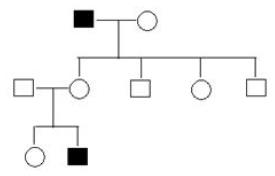Solution:

Since the trait skips a generation , it cannot be a dominant trait. Only the males are affected, so, it has to be an X-linked recessive inheritance.

QUESTION: 6

Match the following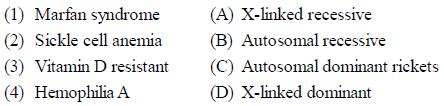Solution:
QUESTION: 7

In a polygenic trait, 4 gene pairs were involved. How many phenotypic classes would you expect in the F2 generation?

Solution:

Phenotypic classes are calculated as 2n+1, where n is the number of gene pairs controlling the trait.

QUESTION: 8

In humans, which of the following is a primary determinant of maleness?

Solution:

In humans, the presence of a Y chromosome results in formation of testis. If the Y chromosome is absent, then ovaries are formed.

QUESTION: 9

Two siblings who inherit 50% of the genome from the mother and 50% from the father show lot of phenotypic differences. Which one of the following events during gametogenesis of the parents will maximally contribute to the difference?

Solution:

According to the Mendelian inheritance theory, during the gametogenesis, the assortment of the genes of one pair is independent to the other pair. Thus, each pair alleles behaves independently and does not influence the segregation of the other. As a result, each gamete receives one member of every pair of alleles in equal frequency with different sets of combinations. Hence, independent assortment results new combinations of character in the offspring showing lot of phenotypic differences in the siblings of the same parent.

QUESTION: 10

In Limnaea peregra, shell coiling is due to maternal effect. If the female parent is dextral (dd) and the male parent is sinistral (dd), the progeny would be

Solution:

Since both the mother and the father have genotype dd, the progeny would contain dd as well. Since the mother did not have a dominant allele, her gametes would not contain the protein required for dextral coiling of the shell in the cytoplasm although she herself had a dextrally coiling shell. Hence, they would all be sinistral.

QUESTION: 11

Blood group type A antigen is a complex oligosaccharide which differs from H antigen present in type O individuals by the presence of terminal

Solution:

IA and IB alleles in human encode glycosyl transferase enzyme, each causing different modification in the terminal sugar of the H-structure (carbohydrate group) present on the surface of RBC. Addition of terminal N- acetylgalactosamine to the H-structure by IA allele results in A antigen and addition of terminal galactose by IB forms B antigen in human RBC, which is absent in the O type individuals.

QUESTION: 12

Following is a hypothetical biochemical pathway responsible for pigmentation of leaves. The pathway is controlled by two independently assorting genes ‘A’ and ‘B’ encoding enzymes as shown below. Mutant alleles ‘a’ and ‘b’ code for non-functional proteins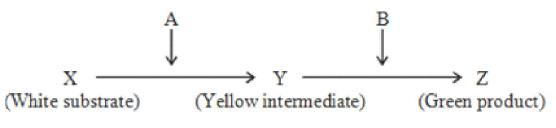What is the expected progeny after selfing a plant with the genotypes AaBb?

Solution:

The above cross can be illustrated as follows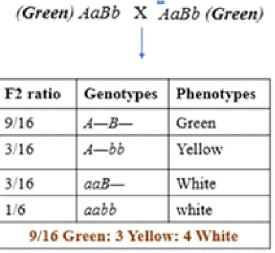*Multiple options can be correct
QUESTION: 13

Which of the following can result in Down Syndrome in an individual?

Solution:

Non-disjunction of chromosome 21 can lead to trisomy of 21 after fertilization with a haploid gamete. This results in Down Syndrome. When a part of chromosome 21 cuts off and gets translocated on chromosome 14, it gives a trisomy like effect when this 14/21 chromosome gets segregated into a gamete and is fertilized. So, both translocation and non-disjunction result in Down Syndrome.

*Multiple options can be correct
QUESTION: 14

X chromosome inactivation

Solution:

Since females contain two X chromosomes, one X chromosome gets inactivated to prevent over-expression of genes in females and to balance the expression in males and females. Since only females contain an extra X chromosome, this happens only in females.

*Multiple options can be correct
QUESTION: 15

In which case will the heterozygous individual (Aa) have a phenotype different from the homozygous dominant individual (aa)?

Solution:

When alleles show a dominant recessive relationship, then the heterozygous and homozygous dominant genotypes show same phenotype. When alleles are co-dominant or incompletely dominant, the heterozygous individuals show different phenotypes such as in ABO blood groups and Antirrhinum flowers.

*Multiple options can be correct
QUESTION: 16

Which of the following is true for polyploidy?

Solution:

Polyploids are sterile because of irregular segregation of chromosomes during meiosis. It may result in aneuploid gametes. The zygotes obtained always die and this inviability explains the sterility of polyploid organisms.

*Multiple options can be correct
QUESTION: 17

Compensation loop is formed when homologues of a chromosome pair. During which of the following events would a  compensation loop form?

Solution:

Deletion and duplication result in an unequal number of alleles on a homologue pair. This results in the formation of a compensation loop in both of these events.

*Answer can only contain numeric values
QUESTION: 18

In a cross between two F1 heterozygous tall plants, the F2 progeny consisted of tall and dwarf plants in the ratio 3 : 1. The probability that out of 7 plants chosen at random, 4 would be tall and 3 would be dwarf ______________ (answer upto 3 decimal points)

Solution:

This is solved using binomial expansion where p = ¾, q = ¼ , n = 7, x = 4. The answer would be 0.173

*Answer can only contain numeric values
QUESTION: 19

In a Drosophila, three recessive mutations can lead to white eyes, yellow body and vestigial wings.  A heterozygous fly is crossed with a fly recessive for all these mutations. Using the following data, the distance between the gene for wing shape and eye color would be ________cM. (Answer upto one point after decimal)

Solution:

q2 = 0.04. Then, q = 0.2. p = 1-q = 0.8.
Frequency of heterozygotes will be 2pq = 2(0.8)(0.2) = 0.32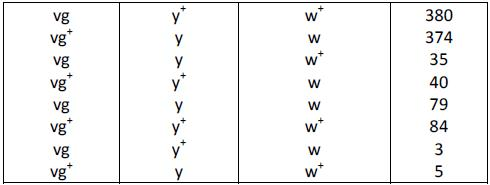The gene w is in the middle. Distance between vg and w will be = Number of SCOs (79+84) + number of DCOs(8) / 1000 = 17.1 cM

*Answer can only contain numeric values
QUESTION: 20

In a population of insects, the color brown (B) is dominant over the color white (b). 4% of all insects are white. The frequency of heterozygous individuals would be _________ (Answer upto two point after decimal)

Solution:

q2 = 0.04. Then, q = 0.2. p = 1-q = 0.8.
Frequency of heterozygotes will be 2pq = 2(0.8)(0.2) = 0.32

*Answer can only contain numeric values
QUESTION: 21

A population is in Hardy-Weinberg equilibrium. If the frequency of a recessive allele for a certain hereditary trait is 0.30. In the next generation percentage of individuals expected to show the dominant trait would be_____%. (Answer in integer)

Solution:

q = 0.30
p = 1–q = 0.70
p2 = 0.70 * 0.70 = 0.49
% of individuals with p2 = 0.49 * 100 = 49%